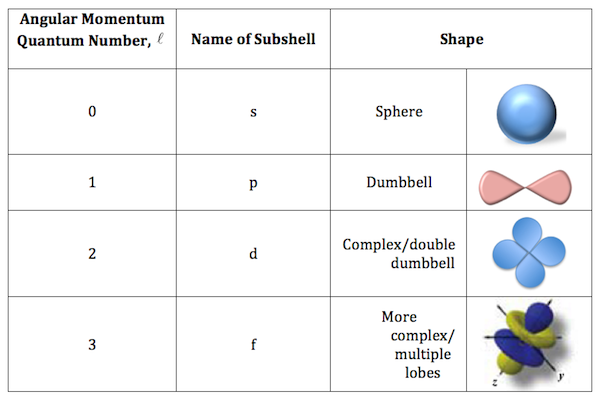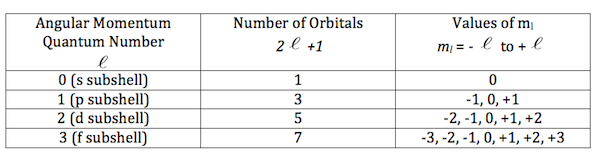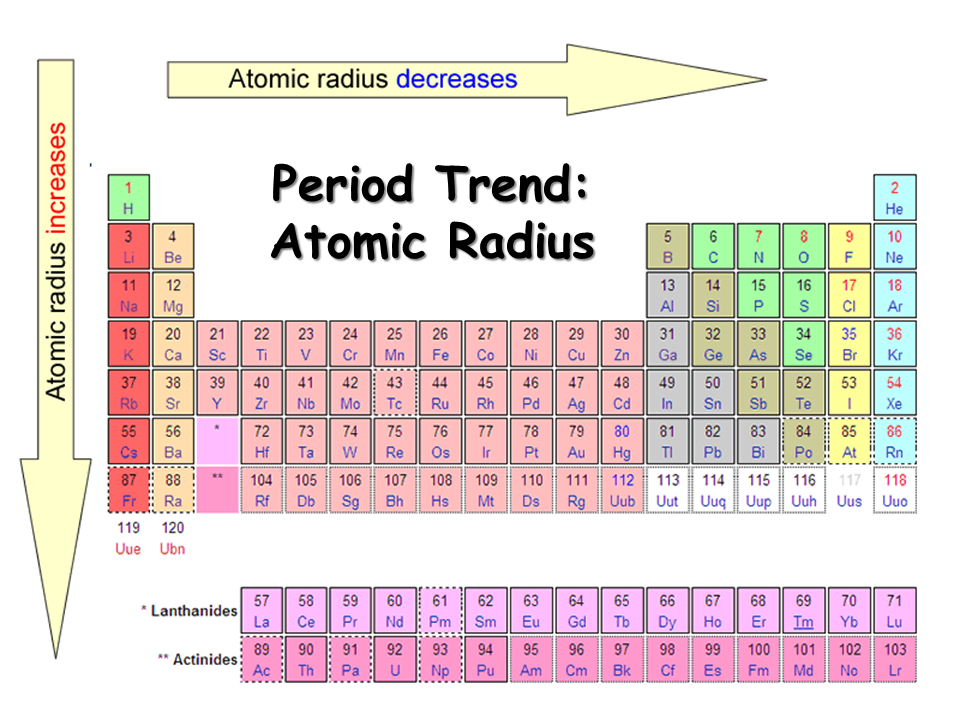Resources:

Have you found videos, websites, or explanations that helped you understand this chapter? Let us know and we'll add them to "Resources" part of this page for other students to use.

Answer Explanations Are Now Available !

Question 1

How many protons does copper have?

Explanationatomic number = protons = electrons

Question 2

How many electrons does copper have? Remember atoms are neutral.

Look Above ^^^^

Question 3

How many neutrons does copper-63 have? Help given in feedback.

Explanation

neutron = atomic mass - atomic number

neutron = 63 - 29 = 34

Question 4

What is the mass of 1 mol of CO2 in g?Explanation

CO2 = 12 + (2 x 16) = 44g

Question 5

What is the mass of 5 molecules of SO3 in g?Explanation

1 mol = 6.02 x 1023 (Avogadro's Number).

5 molecules x ( 1 mol / 6.02 E23) x ( 80g / 1mol ) = 6.64e-22 g

Question 6

Correctly match the element with the property.
 Metal that reacts with cold water to produce OH- and H2(g) Answer 1Choose...SiCrSNaHeMetal (all conduct electricity)--no reaction with water, but reacts with acid to produce H2 gas Answer 2Choose...SiCrSNaHeNon-metal--does not conduct electricity Answer 3Choose...SiCrSNaHeNoble gas--inert, stable electron configuration Answer 4Choose...SiCrSNaHeSemimetal--poor conductor of electricity Answer 5Choose...SiCrSNaHeExplanation

• Na reacts to produce OH- and H2 ions
• Transition metal react with acid to produce H2
• Nonmetal do not conduct electricity
• He is a nobel gas (look at the periodic table)
• Silicon is a common semiconductor/ semimetal

Question 7

What is the correct formula for aluminum sulfide?
Select one:Explanation

Al: +3

S: -2

Now cross the charges to get : Al2S3

Question 8

Which compound is the most ionic?

Select one:Explanation

The molecule with the greatest difference in electronegativity is the most ionic.Question 9

Match properties correctly

 Indicates energy and size of orbital Answer 1Choose...2npsdfl=0 Answer 2Choose...2npsdfl=1 Answer 3Choose...2npsdfl=2 Answer 4Choose...2npsdfl=3 Answer 5Choose...2npsdfNumber of possible electron spins for each orbital Answer 6Choose...2npsdfExplanationEach single orbital can hold a max of 2 electrons

Question 10

Select the correct set of quantum mechanical numbers for an electron in an s, p, d, and f orbital. (4 correct answers.)

Allowed Combination of Quantum Numbers

Select one or more:Explanationmagnetic spin can be only +1/2 or -1/2

Question 11

Correctly match the information for the angular momentum quantum number (l) which gives the shape of the orbital. A nodal plane is a region where the electron density is zero, that is, the probability of finding the electron there is zero.

 The maximum number of electrons in an electron orbital. Answer 1Choose...2dspfSpherically symmetric orbital--no nodal planes Answer 2Choose...2dspfOrbital shaped like a peanut or dumbbell--one nodal plane Answer 3Choose...2dspf4 of the 5 orbital shaped like a double peanut--2 nodal planes Answer 4Choose...2dspfOrbital shaped like flower or fancy--3 nodal planes Answer 5Choose...2dspfExplanationQuestion 12

What is the ground-state electron configuration for S?

Select one:Explanation

Count from left to right.Question 13

What is the electron configuration for S2-?
Select one:Explanation

1s22s22p63s23p4 + 2

1s22s22p63s23p6

Question 14

What is the electron configuration for K1+?
Select one:Explanation

Subtract an electron from the original from highest nth energy level\

1s22s22p63s23p64s1 -1

Question 15

Match atomic radius from largest to smallest.

 K Answer 1Choose...Fourth largest atomic radiusLargest atomic radiusSecond largest atomic radiusSmallest atomic radiusThird largest atomic radiusAl Answer 2Choose...Fourth largest atomic radiusLargest atomic radiusSecond largest atomic radiusSmallest atomic radiusThird largest atomic radiusNa Answer 3Choose...Fourth largest atomic radiusLargest atomic radiusSecond largest atomic radiusSmallest atomic radiusThird largest atomic radiusAr Answer 4Choose...Fourth largest atomic radiusLargest atomic radiusSecond largest atomic radiusSmallest atomic radiusThird largest atomic radiusS Answer 5Choose...Fourth largest atomic radiusLargest atomic radiusSecond largest atomic radiusSmallest atomic radiusThird largest atomic radiusExplanationQuestion 16

Match ionization energies from smallest to largest.

 K Answer 1Choose...Largest ionization energySmallest ionization energyThird smallest ionization energyFourth smallest ionization energySecond smallest ionization energyAl Answer 2Choose...Largest ionization energySmallest ionization energyThird smallest ionization energyFourth smallest ionization energySecond smallest ionization energyNa Answer 3Choose...Largest ionization energySmallest ionization energyThird smallest ionization energyFourth smallest ionization energySecond smallest ionization energyAr Answer 4Choose...Largest ionization energySmallest ionization energyThird smallest ionization energyFourth smallest ionization energySecond smallest ionization energyS Answer 5Choose...Largest ionization energySmallest ionization energyThird smallest ionization energyFourth smallest ionization energySecond smallest ionization energyExplanationQuestion 17

What is the correct formula for iron (III) oxide?
Select one:Explanation

Fe (III)  : +3

O: -2

Cross the charges to get

Fe2O3

Question 18

Which of the following tin (IV) compounds makes sense using oxidation states and conservation of charge?

Oxidation State and Polyatomic Ions

Select one:Tin (IV) :  +4

: -1

Question 19

Warmup question. How many moles of Ca(OH)2 are required to neutralize (react completely with) 0.28 moles of HCl? The balanced reaction is:

2HCl(aq) + Ca(OH)2(aq) → 2H2O(l) + CaCl2(aq)Explanation

0.28 mol HCl x ( 1 mol  Ca(OH)2  /  2 mol HCl) = 0.14

Question 20

What volume in L of 0.42 M Ca(OH)2 is required neutralize 0.29 moles of HCl? The balanced reaction is:

2HCl(aq) + Ca(OH)2(aq) → 2H2O(l) + CaCl2(aq)Explanation

0.29 mol HCl x ( 1 mol Ca(OH)2 / 2 mol HCl ) x ( 1 L / 0.42mol Ca(OH)2) = 0.34

Question 21

What volume in L of chlorine at 30oC and 750 torr is required to react completely with 8.73 g aluminum:

2Al(s) + 3Cl2(g) → 2AlCl3(s)

Calculate the moles chlorine needed (as in the previous problem), then use the ideal gas law--PV = nRT. Additional help is given in the feedback.Explanation

moles Al = 8.73 g / 26.9815 g/mol=0.3236

the ratio between Al and Cl2 is 2 : 3

moles Cl2 required = 0.3236 x (3 / 2) = 0.485
p = 750/760 = 0.987 atm
T = 30 + 273 = 303 K

V = nRT/P = 0.485 x 0.08206 x 303 / .987  = 12.31

Question 22

The following reaction takes place at a certain elevated temperature:

Fe2O3(s) + 3CO(g) → 2Fe(l) + 3CO2(s)

What is the percent yield of iron if 53.6 g Fe2O3 in excess CO produces 18.3 g Fe? The M.W. of Fe2O3 is 159.7 g/mol and the M.W. of CO is 28.01 g/mol.

Recall that the percent yield is the actual yield divided by the theoretical yield times 100%. Additional help is given in the feedback.Explanation

53.6g / 159.7 = .3356 mol Fe2O3

.3356 mol Fe2O3 x ( 2 mol Fe / 1 mol Fe2O3 ) x ( 56g / 1 mol Fe) = 37.59 g

37.59 g (theoretical)

18.3 g (actual)

18.3 / 37.59 = 0.4882 x 100 = 48.82 %Question 23

What is the mass percent of carbon in sugar, C12H22O11? M.W. sugar = 342 g/mol.

Help is given in the feedback.Explanation

C = 12 x 12 = 144

144/ 342 = 0.42 x 100 = 42%

Question 24

Analysis of 3.500 g sample of an unknown compound shows that it contains 1.400 g C, 0.235 g H, and the rest O. What is the empirical formula of this compound?

Hint, given in feedback.
Select one:Explanation

C : 1.400 g / 12 g/mol = 0.1167

H: 0.235 g / 1.008 g/mol = 0.2331

O : 1.865 / 16.00 g/mol = 0.1166

C :  0.1167 / 0.1166 = 1

H : 0.2331 / 0.1166 = 2

O :  0.1166/  0.1166 = 1

Question 25

Hemoglobin has a mass of about 67,000 g/mol. It is 0.3335% iron by mass. How many iron atoms are in a molecule of hemoglobin?

Help is given in the feedback.Explanation

67,000 g/mol x 0.003335 = 223.445 g/mol of iron

223.445g/mol  x  (1/55.85 mol/g ) = 4 atoms